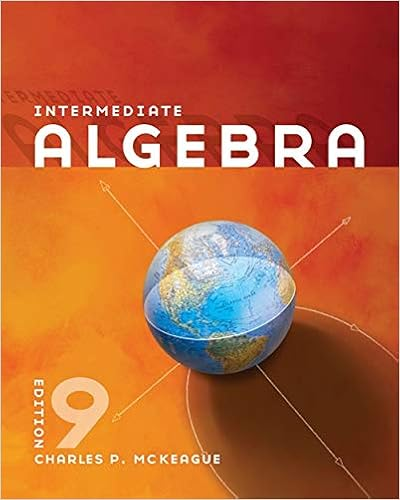# Produces f amount of the good the price it sells the

• Homework Help
• 17
• 100% (2) 2 out of 2 people found this document helpful

This preview shows page 8 - 11 out of 17 pages.

##### We have textbook solutions for you!
The document you are viewing contains questions related to this textbook.The document you are viewing contains questions related to this textbook.
Chapter 6 / Exercise 71
Intermediate Algebra
MckeagueExpert Verified
produces “F” amount of the good, the price it sells the good for (“G”) is greater than the average cost per unit of producing this level of output. Since the price exceeds the ATC at this level of output then the firm must be earning positive economic profit. d) The socially optimal amount of the good is that quantity where the demand curve intersects the MC curve. From the graph we can locate this point and see that we have the following: (Q, P) = (E, 5). Let’s use the demand curve equation to solve for the value of “E”:
##### We have textbook solutions for you!
The document you are viewing contains questions related to this textbook.The document you are viewing contains questions related to this textbook.
Chapter 6 / Exercise 71
Intermediate Algebra
MckeagueExpert Verified
9 P = 30 – (1/5)Q but Q = E in this case, so P = 30 – (1/5)E 5 = 30 – (1/5)E E = 125 units of output Here’s the amended graph where the shaded area shows the total subsidy that the monopolist must receive if they are to produce the socially optimal amount of the good, “E”. e) If the monopolist is regulated by AC pricing regulation the monopolist will produce where P = ATC and this will occur at that quantity where the LRATC curve intersects the demand curve. In the provided graph we can see that this is at a quantity of 90 units. We can find the regulated price by using this quantity in the market demand curve: thus, P = 30 – (1/5)(Q) where Q is set to 90 units, the quantity where the firm will break even. P = 30 – (1/5)(90) P = 12 Here’s the amended graph: 5. Consider a market that is served by a single producer. This market has significant barriers to entry so the single producer has market power and is not likely to face any competition due to these barriers of entry. You are given the following information about this market: Market Demand: Q = 450 – 3P MC = 30 Fixed Cost for the Producer: FC = 50
•••